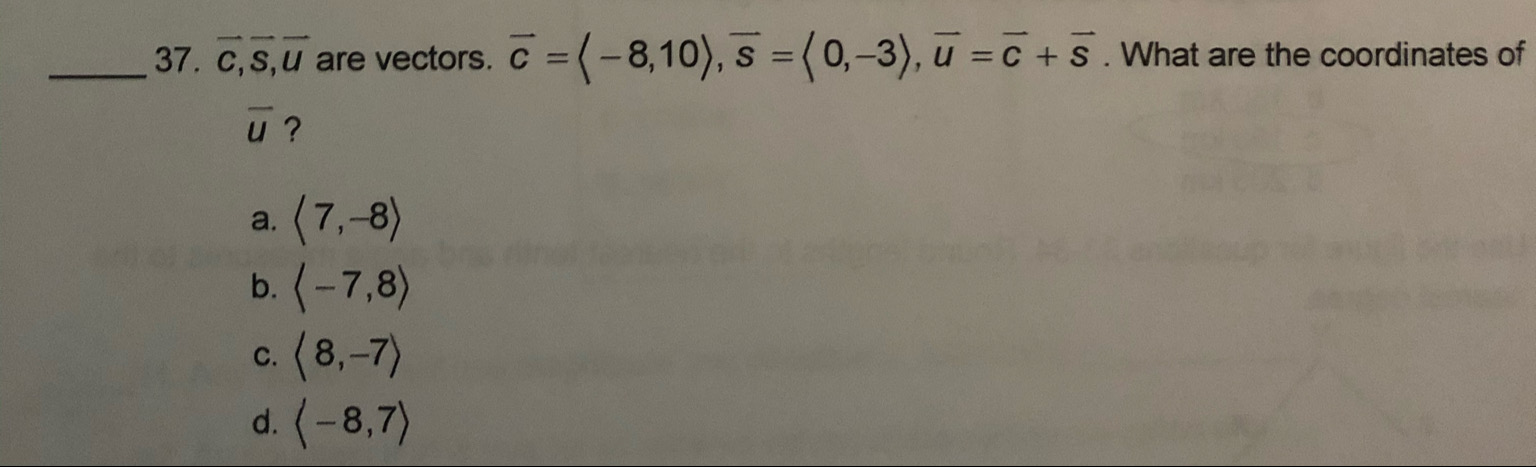# 37. C,s,u are vectors. c =(-8,10), s =(0,-3)), u =c + s . What are the coordinates ofu ?a. (7,-8)b.-7,8)c. (8,-7)d. (-8,7)

Question
1 viewshelp_outlineImage Transcriptionclose37. C,s,u are vectors. c =(-8,10), s =(0,-3)), u =c + s . What are the coordinates of u ? a. (7,-8) b.-7,8) c. (8,-7) d. (-8,7) fullscreen
check_circle

Step 1

The given vect...

### Want to see the full answer?

See Solution

#### Want to see this answer and more?

Solutions are written by subject experts who are available 24/7. Questions are typically answered within 1 hour.*

See Solution
*Response times may vary by subject and question.
Tagged in
MathGeometry

### Other